# Lens space

(diff) ← Older revision | Latest revision (diff) | Newer revision → (diff)
A manifold of odd dimension that arises as the orbit space of the isometric free action of a cyclic groupon the sphere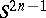(cf. Action of a group on a manifold). It is convenient to take forthe unit sphere in the complex spacein which a basis is fixed. Suppose thatacts on each coordinate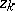by multiplying it by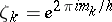, where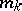is invertible modulo, that is, there are numbers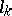such that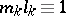(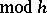). This specifies an isometric free (thanks to the condition thatis invertible) action of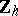on, and any such action has this form described in a suitable coordinate system. The Reidemeister torsion corresponding to an-th root of unityis defined for a lens space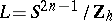constructed in this way by the formula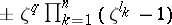. Any piecewise-linear lens space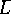homeomorphic to it must have equal (up to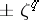) torsion, and it turns out that the sets of numbers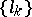and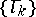must coincide. Thus, these sets characterize lens spaces uniquely up to a piecewise-linear homeomorphism and even up to an isometry; on the other hand, by the topological invariance of the torsion, they also characterize lens spaces uniquely up to a homeomorphism. A lens space is aspherical up to dimension(that is,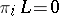,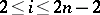), and the fundamental group is equal toin view of the fact that the sphereis the universal covering for. The homology ofcoincides up to dimensionwith the homology of the group, that is, it is equal toin all dimensions fromto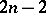and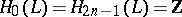. The direct limit of the spacesgives an Eilenberg–MacLane space of type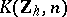. Two lens spaces are homotopy equivalent if and only if the linking coefficients (cf. Linking coefficient)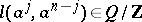coincide, whereis a generator of the two-dimensional cohomology group. By means of these invariants one can establish the existence of asymmetric manifolds among lens spaces.
In the three-dimensional case lens spaces coincide with manifolds that have a Heegaard diagram of genus 1, and so they are Seifert manifolds (cf. Seifert manifold). It is convenient to represent the fundamental domain of the action ofon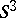as a "lens" , i.e. the union of a spherical segment and its mirror image; this is how the name lens surface arose.